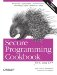# Testing Whether a String Contains a Valid Number

Problem

You have a string and you need to find out if it contains a valid number.

Solution

You can use the Boost lexical_cast function template to test for a valid number. Using this approach, a valid number can include a preceding minus sign, or a preceding plus sign, but not whitespace. I give a few examples of the kinds of formats that work with lexical_cast in Example 3-5.

Example 3-5. Validating a string number

```#include
#include

using namespace std;
using boost::lexical_cast;

template
bool isValid(const string& num) {

bool res = true;

try {
T tmp = lexical_cast(num);
}
res = false;
}

return(res);
}

void test(const string& s) {

if (isValid(s))
cout << s << " is a valid integer." << endl;
else
cout << s << " is NOT a valid integer." << endl;

if (isValid(s))
cout << s << " is a valid double." << endl;
else
cout << s << " is NOT a valid double." << endl;

if (isValid(s))
cout << s << " is a valid float." << endl;
else
cout << s << " is NOT a valid float." << endl;
}

int main( ) {

test("12345");
test("1.23456");
test("-1.23456");
test(" - 1.23456");
test("+1.23456");
test(" 1.23456 ");
test("asdf");
}```

Here's the output from this example:

```12345 is a valid integer.
12345 is a valid double.
12345 is a valid float.
1.23456 is NOT a valid integer.
1.23456 is a valid double.
1.23456 is a valid float.
-1.23456 is NOT a valid integer.
-1.23456 is a valid double.
-1.23456 is a valid float.
- 1.23456 is NOT a valid integer.
- 1.23456 is NOT a valid double.
- 1.23456 is NOT a valid float.
+1.23456 is NOT a valid integer.
+1.23456 is a valid double.
+1.23456 is a valid float.
1.23456 is NOT a valid integer.
1.23456 is NOT a valid double.
1.23456 is NOT a valid float.
asdf is NOT a valid integer.
asdf is NOT a valid double.
asdf is NOT a valid float.```

Discussion

The lexical_cast function template converts a value from one type to another. It is declared like this:

```template
Target lexical_cast(Source arg)```

Source is the type of the original variable, and Target is the type of the variable being converted to. So, for example, if you want to convert from a string to an int, you invoke lexical_cast like this:

`int i = lexical_cast(str); // str is a string`

lexical_cast does the parsing and attempts the conversion. If the conversion is not possible, it throws a bad_lexical_cast exception. In Example 3-5, I only want to test for validity and don't need to keep the destination variable around, so I return true if no exception is thrown, false otherwise.

You only have to supply the first template argument to lexical_cast because it's a function template, which means the compiler can deduce the type of the function argument and use that as the second template argument. Explaining this distinction is more confusing than illustrating it, so let me use a code example. Instead of invoking lexical_cast as in the previous code snippet, you could do this:

`int i = lexical_cast(str);`

This means the same thing, but you don't have to supply the string argument because the compiler can see that str is a string and figure out the rest.

If you are going to write a similar wrapper function to test for validity and return true or false, you would do well to write it as a function template. This way, you only have to write it once with a parameterized type, and a different version will be instantiated each time you use it on a different type.

lexical_cast is also handy for converting from one numeric type to another; I discuss more about that in Recipe Recipe 3.6.

Recipe 3.6Secure Programming Cookbook for C and C++: Recipes for Cryptography, Authentication, Input Validation & More
ISBN: 0596003943
EAN: 2147483647
Year: 2006
Pages: 241